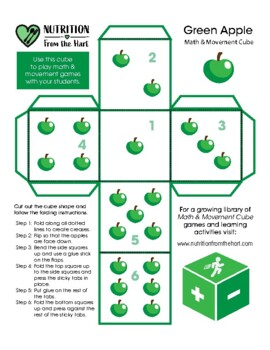# Math & Movement Cube: Nutrition Education Starter PackK - 4th
Subjects
Standards
Resource Type
Formats Included
• PDF

### Description

This is the first Math & Movement Cube pack in what will be a series of fun instructional tools. This pack is designed to help you reinforce math skills while normalizing healthy food choices, celebrating the amazing apple, and providing much needed classroom activity boosts. Complete as a class, individually, or in math centers. Look for expanded packs coming soon.

Total Pages
N/A
Teaching Duration
N/A
Report this resource to TpT
Reported resources will be reviewed by our team. Report this resource to let us know if this resource violates TpT’s content guidelines.

### Standards

to see state-specific standards (only available in the US).
Use addition and subtraction within 20 to solve word problems involving situations of adding to, taking from, putting together, taking apart, and comparing, with unknowns in all positions, e.g., by using objects, drawings, and equations with a symbol for the unknown number to represent the problem.
Use addition to find the total number of objects arranged in rectangular arrays with up to 5 rows and up to 5 columns; write an equation to express the total as a sum of equal addends.
Interpret products of whole numbers, e.g., interpret 5 × 7 as the total number of objects in 5 groups of 7 objects each. For example, describe a context in which a total number of objects can be expressed as 5 × 7.
Use multiplication and division within 100 to solve word problems in situations involving equal groups, arrays, and measurement quantities, e.g., by using drawings and equations with a symbol for the unknown number to represent the problem.
Add within 100, including adding a two-digit number and a one-digit number, and adding a two-digit number and a multiple of 10, using concrete models or drawings and strategies based on place value, properties of operations, and/or the relationship between addition and subtraction; relate the strategy to a written method and explain the reasoning used. Understand that in adding two-digit numbers, one adds tens and tens, ones and ones; and sometimes it is necessary to compose a ten.

### Questions & Answers

Teachers Pay Teachers is an online marketplace where teachers buy and sell original educational materials.